# 二次方程 实例• 描述实际情况和写下方程
• 解！
• 用常理来分析结果## 球、箭、导弹和石头

……二次方程 可以告诉你球在任何时间的位置！

## 例子：抛球

### 你把球垂直向上抛，球离开你的手时离地3m，速度14 m/s。球在什么时候掉到地上？

（注意：t 是时间，以秒为单位）

 开始时高度是3m： 3 球以每秒14米（14 m/s）的速度向上飞： 14t 地心引力把球向下拉，把它的高度以 大约 5 米每平方秒 （m/s2） 的率减少： −5t2 （若你有兴趣：-5t2 是从 -(½)at2，a=9.8 m/s2 简化而来）

h = 3 + 14t − 5t2

3 + 14t − 5t2 = 0

−5t2 + 14t + 3 = 0

 把所有项乘以 −1 会简单点： 5t2 − 14t − 3 = 0 然后去分解因式。 我们用二次式因式分解的方法："找两个数，要它们的积是 a×c，它们的和是 b"。 a×c = −15，b = −14. −15 的因数是：−15、−5、−3、−1、1、3、5、15 试试不同的组合，我们找到 −15 和 1： (−15×1 = −15, −15+1 = −14) 以 −15 和 1 重写中间部分： 5t2 − 15t + t − 3 = 0 因式分解头两项和后两项： 5t(t − 3) + 1(t − 3) = 0 公因式是 (t − 3)： (5t + 1)(t − 3) = 0 两个解是： 5t + 1 = 0 或 t − 3 = 0 t = −0.2  或  t = 3

"t = −0.2" 是负时间，在这例子里是不可能的。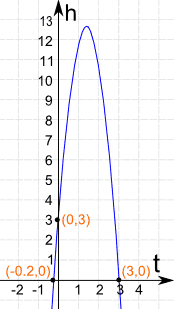(0,3) 当 t=0 （开始）时球在 3m 高

(−0.2,0) 说在球离手前 −0.2秒球是在地上（高度为零）。这是不对的。按常理，我们不管它。

(3,0) 的意思是球离手后 3秒球又在地上了。

−b/2a求最高点在水平（时间）轴上的值：

• t = −b/2a = −(−14)/(2 × 5) = 14/10 = 1.4 秒

• h = −5t2 + 14t + 3 = −5(1.4)2 + 14 × 1.4 + 3 = 12.8米
• 离手1.4秒后，球到达12.8米的最高点。## 例子：新运动自行车

你设计了一款新运动自行车！

你打算卖它个上千上万辆来淘你的第一桶金！

你的成本是：

• \$700,000 来准备生产、广告开支等等
• \$110 来制造一辆运动自行车

（你要卖到国际市场，所以一切用美金来算！）你做的市场研究告诉你，售量 会像这个 "需求曲线"：

• 售量 = 70,000 − 200P

其中 "P" 是每辆的价钱

例如，如果价钱是：

• \$0，你送给人 70,000辆自行车了
• \$350，你一辆也卖不去
• \$300，你可以卖 70,000 − 200×300 = 10,000

好了……最好（赚的最多）的价钱是多少？还有，你应该制造多少辆？

我们来制定一些方程！

可以卖多少辆要看每辆的价钱，所以我们用价钱 "P" 为变量

• 销量 = 70,000 − 200P
• 销售额（美元） = 销量 × 单价 = (70,000 − 200P) × P = 70,000P − 200P2
• 成本 = 700,000 + 110 x (70,000 − 200P) = 700,000 + 7,700,000 − 22,000P = 8,400,000 − 22,000P
• 利润 = 销售额-成本 = 70,000P − 200P2 − (8,400,000 − 22,000P) = −200P2 + 92,000P − 8,400,000

利润 = −200P2 + 92,000P − 8,400,000

对了，是个二次方程。这次我们用 配方法来解。

### 解：−200P2 + 92,000P − 8,400,000 = 0

一、所有项除以 -200

P2 – 460P + 42000 = 0

二、把数字项移到右边：

P2 – 460P = -42000

三、左边配方，把等值加到右边以保持平衡：

(b/2)2 = (−460/2)2 = (−230)2 = 52900

P2 – 460P + 52900 = −42000 + 52900
(P – 230)2 = 10900

四、每边取平方根：

P – 230 = ±√10900 = ±104 （舍入到最近的整数）
五、每边减 (-230) （就是每边加 230）：

P = 230 ± 104 = 126 或 334

这告诉我们什么？当价钱是 \$126 或 \$334 时利润是零。

可是我们想知道的是最大利润……

刚好在这两个价钱的正中间！\$230

图是这样：利润 = −200P2 + 92,000P − 8,400,000

最好的价钱是 \$230，在这个卖价，情形将会是：

• 销量 = 70,000 − 200 x 230 = 24,000
• 销售额 = \$230 x 24,000 = \$5,520,000
• 利润 = \$5,520,000 − \$3,340,000 = \$2,180,000

相当可观！

## 例子：小钢框架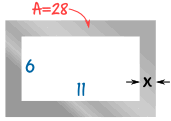你的公司将会制造框架作为一个产品的部件。

我们会从一块矩形的钢片的正中间切出一个矩形的空间，剩下来的便是我们想要的框架。我们需要框架里的钢的面积是 28 cm2

框架内部的空间的大小是 11 cm × 6 cm

框架每边的宽度 x 是多少？

钢片原来（未切前）的面积：

面积 = (11 + 2x) × (6 + 2x) cm2
面积 = 66 + 22x + 12x + 4x2
面积 = 4x2 + 34x + 66

切出中间（11 × 6 的矩形）之后，剩下来的钢的面积：

面积 = 4x2 + 34x + 66 − 66
面积 = 4x2 + 34x### 我们现在用 图 来解这个！

这是 4x2 + 34x 的图：

我们用一条水平先来显示想要的面积，28

在以下的情况下面积是 28 cm2

x 是大约 −9.3 或 0.8

不可能有负值的宽度（x），所以答案是：

x = 0.8 cm （大约）

## 例子：江河畅游

### 一个三小时的江河畅游旅程的速度是向上游行驶15km，然后掉头回程。河流的速度是每小时 2 km。邮轮的速度是多少及上游旅程用了多少时间？我们要考虑两个速度：邮轮在水里的速度和邮轮相对于陆地的速度：

• x = 邮轮在水里的速度(km/h)
• v = 邮轮相对于陆地的速度（km/h）

因为河流向下游的速度是 2 km/h：

• 溯流时，v = x−2（速度减慢了 2 km/h）
• 顺流时，v = x+2（速度加快了 2 km/h）

我们可以把速度转换为时间：

时间 = 距离 / 速度

（以 4 km/h 的速度走 8 km 需要 8/4 = 2小时，对不对？）

旅程总共用了 3 小时：

总时间 = 向上游时间 + 向下游时间 = 3 小时

全部集合在一起：

总时间 = 15/(x−2) + 15/(x+2) = 3 小时

用代数来解 "x"。

乘以 (x-2)(x+2) 来移除分数：

3(x-2)(x+2) = 15(x+2) + 15(x-2)

展开：

3(x2−4) = 15x+30 + 15x−30

移到左边，再简化：

3x2 − 30x − 12 = 0

这是个二次方程！这一次我们用 二次公式 来解：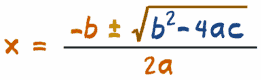其中 abc
二次方程的 "一般形式" 里的系数：ax2 + bx + c = 0

### 解 3x2 - 30x - 12 = 0

 系数是： a = 3, b = −30 and c = −12 二次公式： x = [ −b ± √(b2−4ac) ] / 2a 代入 a、b 和 c： x = [ −(−30) ± √((−30)2−4×3×(−12)) ] / (2×3) 解： x = [ 30 ± √(900+144) ] / 6 x = [ 30 ± √(1044) ] / 6 x = ( 30 ± 32.31 ) / 6 x = −0.39 或 10.39

答案： x = −0.39 10.39 （保留2位小数）

x =−0.39 不合理，但 b = 10.39 就对了！

答案：邮轮的速度 = 10.39 km/h （保留2位小数）

所以向上游的时间 = 15 / (10.39−2) = 1.79 小时 = 1 小时 47分

向下游的时间 = 15 / (10.39+2) = 1.21 小时 = 1 小时 13分

## 例子：并联电阻

两个电阻并联，如图：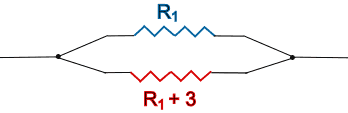并联后的电阻值为 2欧姆，其中一个电阻的电阻值比另一个大 3欧姆。

每个电阻的电阻值为多少？

并联电阻值 "RT" 的公式是：

1RT   =   1R1 + 1R2

在这例子里，RT = 2，R2 = R1 + 3

1 2   =   1 R1 + 1 R1+3

好，我们来解它：

 把所有项乘以 2R1(R1 + 3) 来移除分数： 2R1(R1+3)2 = 2R1(R1+3)R1 + 2R1(R1+3)R1+3 再简化： R1(R1 + 3) = 2(R1 + 3) + 2R1 展开： R12 + 3R1 = 2R1 + 6 + 2R1 把项移到左边： R12 + 3R1 − 2R1 − 6 − 2R1 = 0 简化： R12 − R1 − 6 = 0

真巧！（为什么总是这么巧？）又是二次方程！

不用绞脑汁了，我们用 二次方程解算器 来解。

• 输入 1、−1 和 −6
• 你应该得到 −2 和 3

R1 不能是负数，所以答案是 R1 = 3欧姆

两个电阻的电阻值分别是 3欧姆和 6欧姆。

## 其他

二次方程在很多领域都很有用：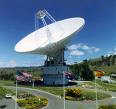抛物面镜面或抛物面天线反射器的形状是以二次方程规定的。

研究透镜和反射镜时也会用到二次方程。

很多牵涉到时间、距离和速度的问题都需要用二次方程来解。